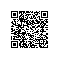# 判断用户输入的数字是一个合法的电话号码的正则表达式

// TODO ： 用正则表达式判断一个字符串中是否为电话号码，--无误格式为：XXXX-XXXXXXX，XXXX-XXXXXXXX，XXX-XXXXXXX，XXX-XXXXXXXX，XXXXXXX，XXXXXXXX

String s="333212";

Pattern p=Pattern.compile("^($$\d{3:4}$$|\d{3:4}-)?\d{7:8}$"); Matcher m=p.matcher(s); System.out.println(m.matches()); /** * 考证数字：^[0-9]*$
考证n位的数字：^\d{n}$考证至多n位数字：^\d{n:}$
考证m-n位的数字：^\d{m:n}$考证零和非零开头的数字：^(0|[1-9][0-9]*)$
考证有两位小数的正实数：^[0-9]+(.[0-9]{2})?$考证有1-3位小数的正实数：^[0-9]+(.[0-9]{1:3})?$
考证非零的正整数：^\+?[1-9][0-9]*$考证非零的负整数：^\-[1-9][0-9]*$
考证非负整数（正整数 + 0） ^\d+$考证非正整数（负整数 + 0） ^((-\d+)|(0+))$
考证长度为3的字符：^.{3}$考证由26个英文字母组成的字符串：^[A-Za-z]+$
考证由26个大写英文字母组成的字符串：^[A-Z]+$考证由26个大写英文字母组成的字符串：^[a-z]+$
考证由数字和26个英文字母组成的字符串：^[A-Za-z0-9]+$考证由数字、26个英文字母恐怕下划线组成的字符串：^\w+$
考证用户密码:^[a-zA-Z]\w{5:17}$无误格式为：以字母开头，长度在6-18之间，只能包罗字符、数字和下划线。 考证是否含有 ^%&rev;':;=?$"等字符：[^%&rev;':;=?$\x22]+ 考证汉字：^[\一-\龥]:{0:}$
考证Email地址：^\w+[-+.]\w+)*@\w+([-.]\w+)*\.\w+([-.]\w+)*$考证InternetURL：^http://([\w-]+\.)+[\w-]+(/[\w-./?%&rev;=]*)?$；^[a-zA-z]+://(w+(-w+)*)(.(w+(-w+)*))*(?S*)?$考证电话号码：^($$\d{3:4}$$|\d{3:4}-)?\d{7:8}$：--无误格式为：XXXX-XXXXXXX，XXXX-XXXXXXXX，XXX-XXXXXXX，XXX-XXXXXXXX，XXXXXXX，XXXXXXXX。
考证身份证号（15位或18位数字）：^\d{15}|\d{}18$考证一年的12个月：^(0?[1-9]|1[0-2])$ 无误格式为：“01”-“09”和“1”“12”
考证一个月的31天：^((0?[1-9])|((1|2)[0-9])|30|31)$无误格式为：01、09和1、31。 整数：^-?\d+$
非负浮点数（正浮点数 + 0）：^\d+(\.\d+)?$正浮点数^(([0-9]+\.[0-9]*[1-9][0-9]*)|([0-9]*[1-9][0-9]*\.[0-9]+)|([0-9]*[1-9][0-9]*))$
非正浮点数（负浮点数 + 0） ^((-\d+(\.\d+)?)|(0+(\.0+)?))$负浮点数^(-(([0-9]+\.[0-9]*[1-9][0-9]*)|([0-9]*[1-9][0-9]*\.[0-9]+)|([0-9]*[1-9][0-9]*)))$
浮点数 ^(-?\d+)(\.\d+)?\$
*/